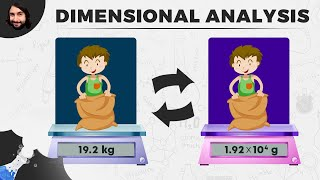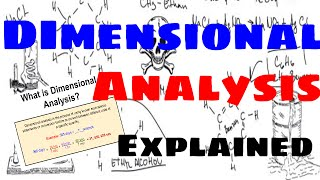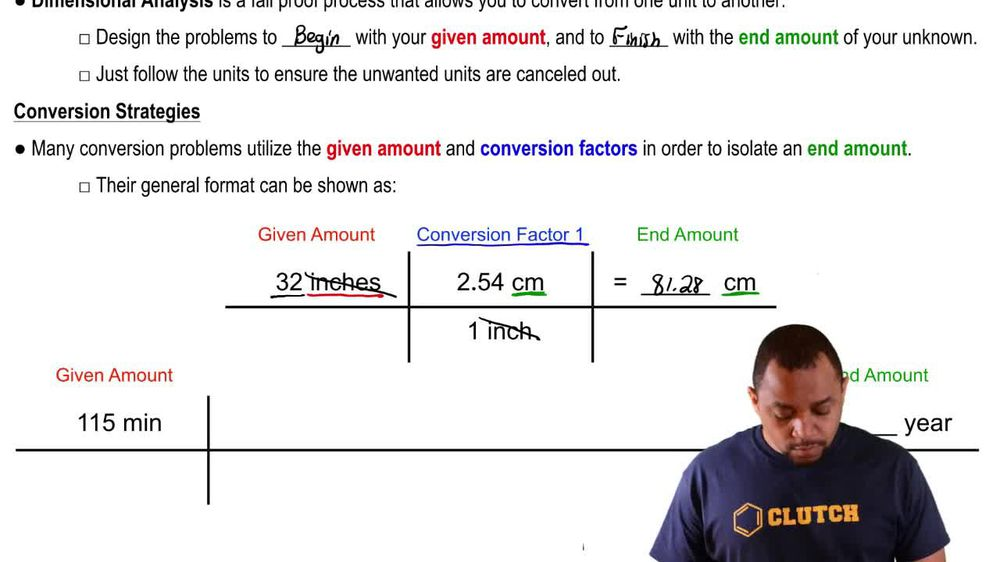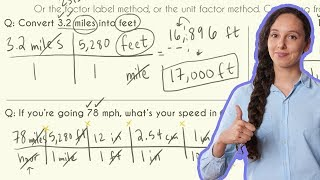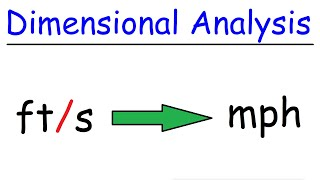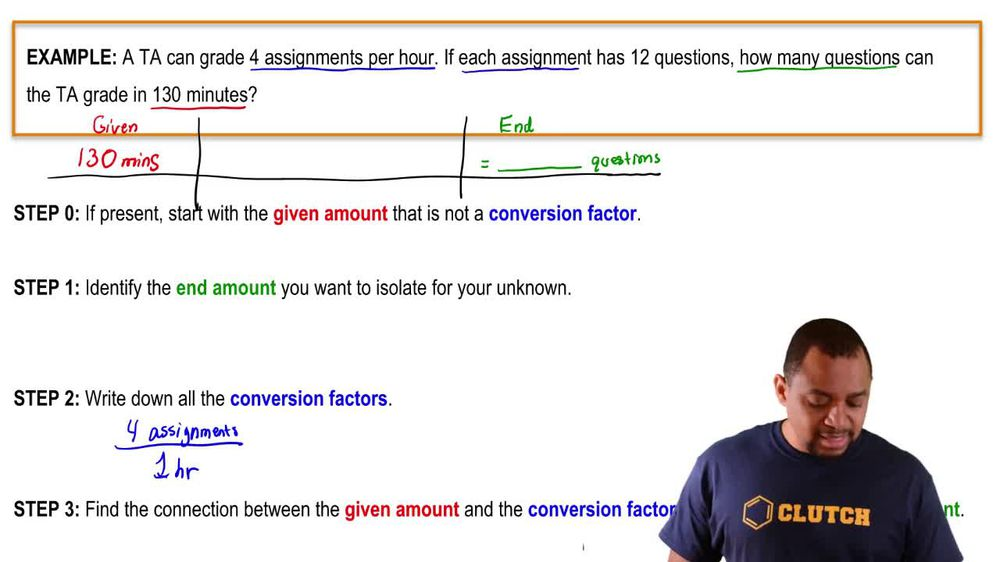Start typing, then use the up and down arrows to select an option from the list.
1. 1. Intro to General Chemistry2. Dimensional Analysis
Problem

# A runner wants to run 10.0 km. Her running pace is 7.5 mi per hour. How many minutes must she run?

Relevant Solution1m
Play a video:
Hey everyone. So here we're told a runner can run 8.9 mph If she wants to run 50 km, how long should she run? Alright, so here we basically have two values being given to us 50 km and then 8.9 mph. Remember always start out with the value that has only one unit attached to it. We're gonna start out with the 50 km easier to manipulate. Here, we have 8.9 MPH, so we're gonna have to find a connection between kilometers and miles. So we're gonna say here that the conversion factor is that one km is .6-1371 mi. Now that we have miles we can bring in The 8.9 miles, so 8.9 miles per one hour, that's how fast you can run miles, cancel out now we have ours And now that we simply have ours, we just changed those in two minutes. One hour is equal to 60 minutes. So that comes out to be 209.45 minutes. Here, we want to round up and become 2 10, 210 minutes, approximately which gives me option B as the correct answer